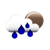### Percentage Change Calculator

 Example: What is the percentage change from 10 to 20? Anwser: ((20 - 10) ÷ 10) × 100 = 100% What is the percentage change from to ? Answer: %

The Percentage Change Calculator online tool enables you to calculate the percentage change between two values like the increase (or decrease) of the price of a product.

#### How to Calculate Percentage Change

You can use the percent change formula to find percentage change of two numbers:

Percentage Change
=
New Number - Original Number / Original Number
× 100

Example: What is the percentage change of 150 to 180 ?

Percentage(%)
=
180 - 150 / 150
× 100

Anwser: 20%

To know how to find percentage increase between two numbers? Please use our percentage increase calculator (or percentage change calculator).

#### References

More references for Percentage Change Calculation

#### Local Weather Status

Ashburn, United States
9th December, 2019 Monday
Light Rain6.67 - 10
Humidity: 93 %
Wind: 6.2 km/h